Ex 10.1

Chapter 10 Class 11 Straight Lines
Serial order wise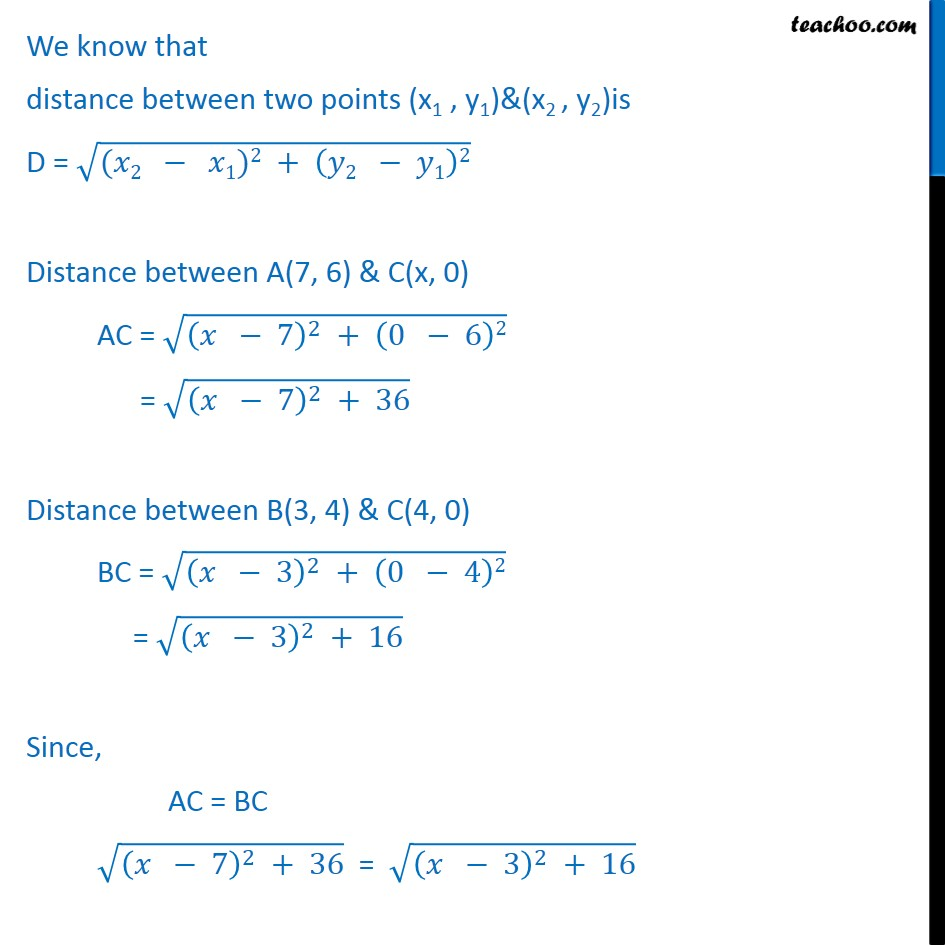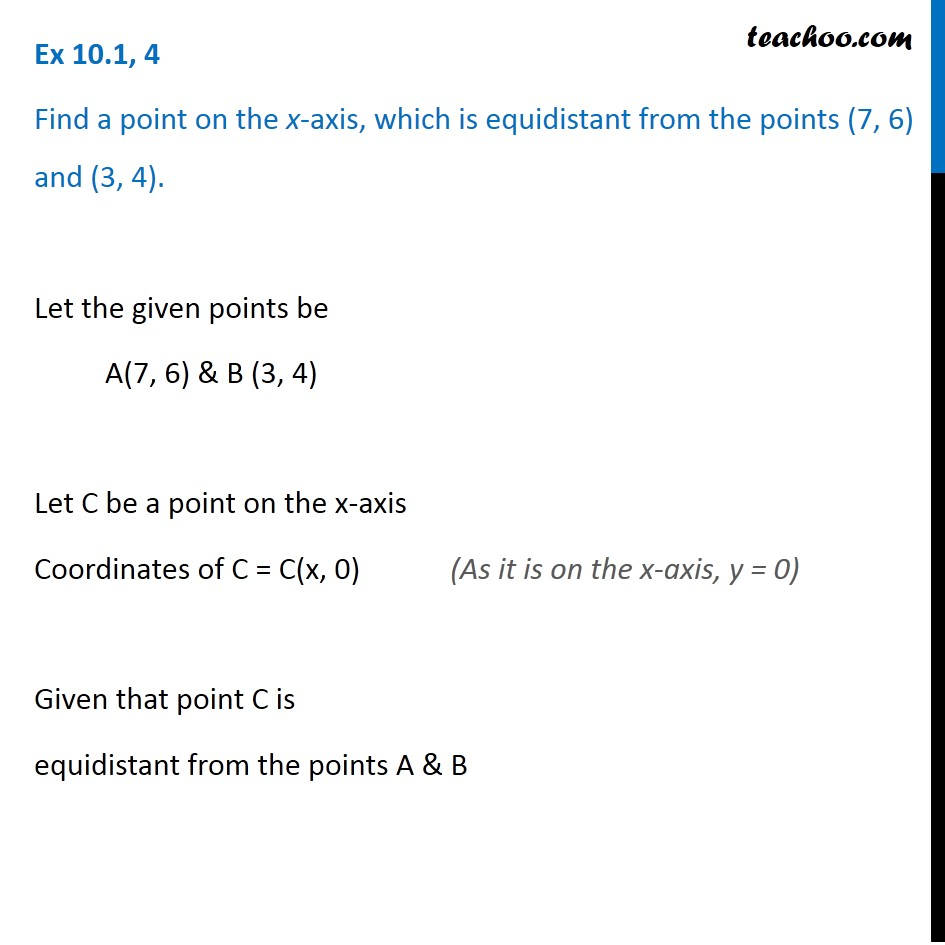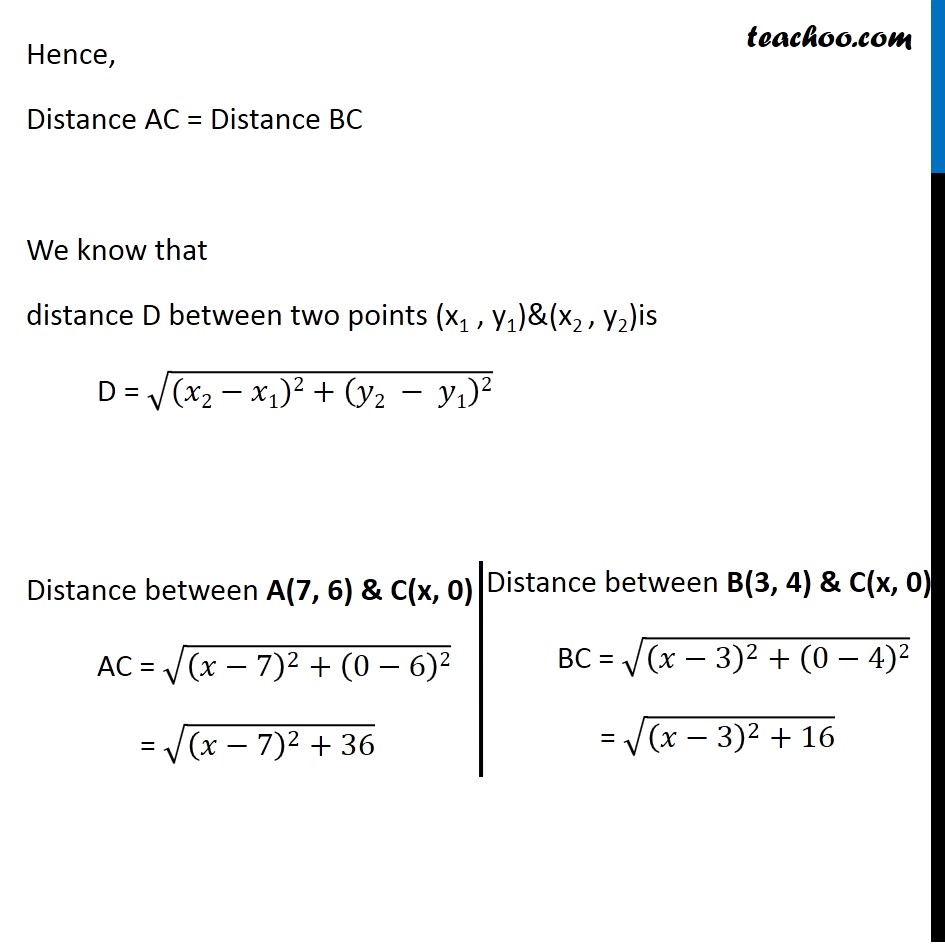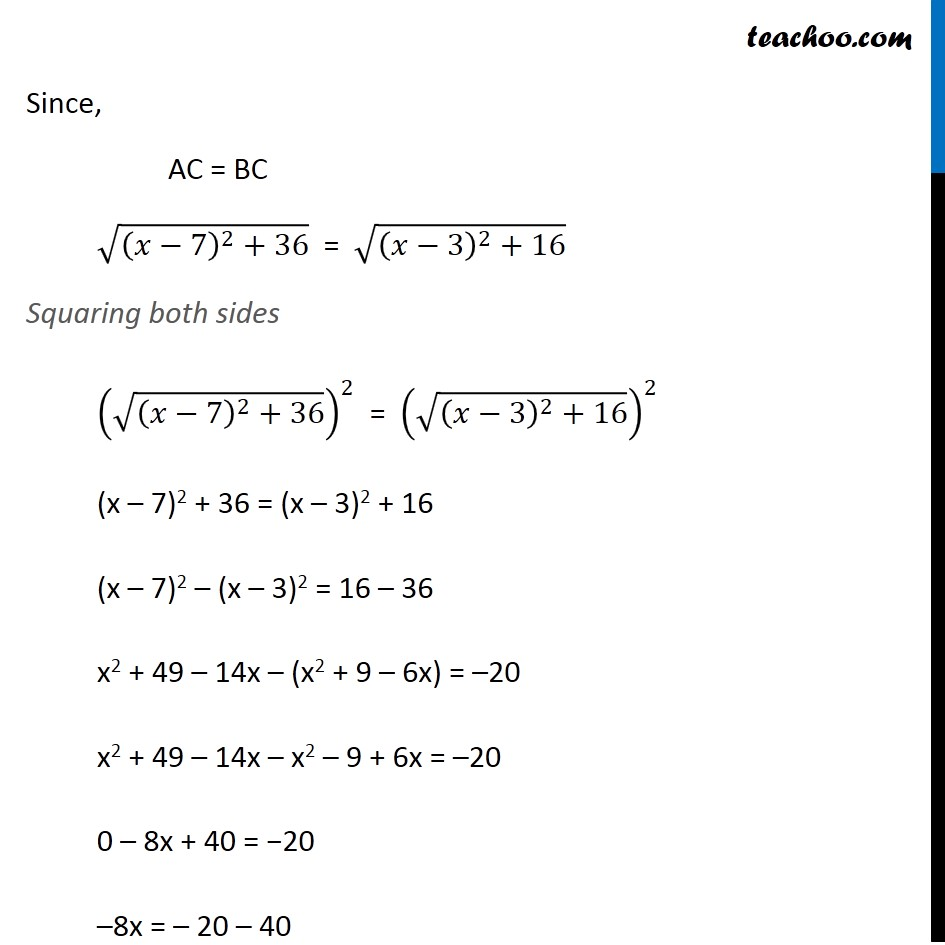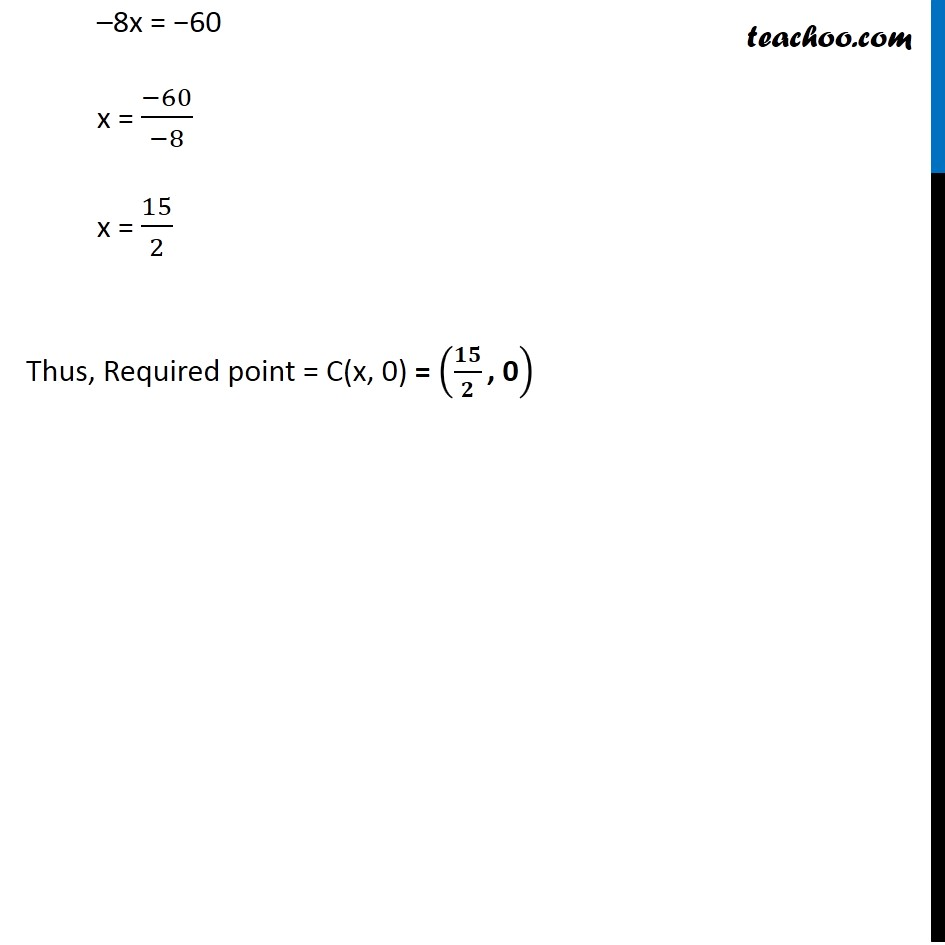Get live Maths 1-on-1 Classs - Class 6 to 12

### Transcript

Ex 10.1, 4 Find a point on the x-axis, which is equidistant from the points (7, 6) and (3, 4). Let the given points be A(7, 6) & B (3, 4) Let C be a point on the x-axis Coordinates of C = C(x, 0) Given that point C is equidistant from the points A & B (As it is on the x-axis, y = 0) Hence, Distance AC = Distance BC We know that distance D between two points (x1 , y1)&(x2 , y2)is D = √((𝑥2−𝑥1)2+(𝑦2 − 𝑦1)2) Distance between A(7, 6) & C(x, 0) AC = √((𝑥−7)^2+(0−6)2) = √((𝑥−7)^2+36) Distance between B(3, 4) & C(x, 0) BC = √((𝑥−3)^2+(0−4)2) = √((𝑥−3)^2+16) Since, AC = BC √((𝑥−7)^2+36) = √((𝑥−3)^2+16) Squaring both sides (√((𝑥−7)^2+36))^2 = (√((𝑥−3)^2+16))^2 (x – 7)2 + 36 = (x – 3)2 + 16 (x – 7)2 – (x – 3)2 = 16 – 36 x2 + 49 – 14x – (x2 + 9 – 6x) = –20 x2 + 49 – 14x – x2 – 9 + 6x = –20 0 – 8x + 40 = −20 –8x = – 20 – 40 –8x = −60 x = (−60)/(−8) x = 15/2 Thus, Required point = C(x, 0) = (𝟏𝟓/𝟐 ", 0" )